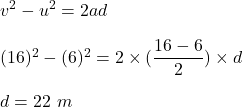## A body moves with constant acceleration. It’s velocity increases from 6 m/s to 16 m/s in 2 seconds. The distance moved by the body in these

Question

A body moves with constant acceleration. It’s velocity increases from 6 m/s to 16 m/s in 2 seconds. The distance moved by the body in these 2 seconds is

in progress 0
5 months 2021-09-01T03:36:57+00:00 1 Answers 5 views 0

The distance moved by the body in these 2 seconds is 22 meters.

Explanation:

Given that,

Initial velocity of a body, u = 6 m/s

Final velocity of the body, v = 16 m/s

Time, t = 2 s

Let d is the distance moved by the body in these 2 seconds is. Using third equation of motion to find it such that,So, the distance moved by the body in these 2 seconds is 22 meters. Hence, this is the required solution.